# Design S-Band Monopulse Tracking RADAR Antenna

This example shows the integration of a 2-by-4 Microstrip patch array and a Rat-race coupler to implement a monopulse operation.

The system is designed for an operating frequency of 3 GHz and the simulated and measured results are compared in this example. The maximum gain of 15.3 dB is achieved at the sum port.

Below diagram shows the top view of the antenna.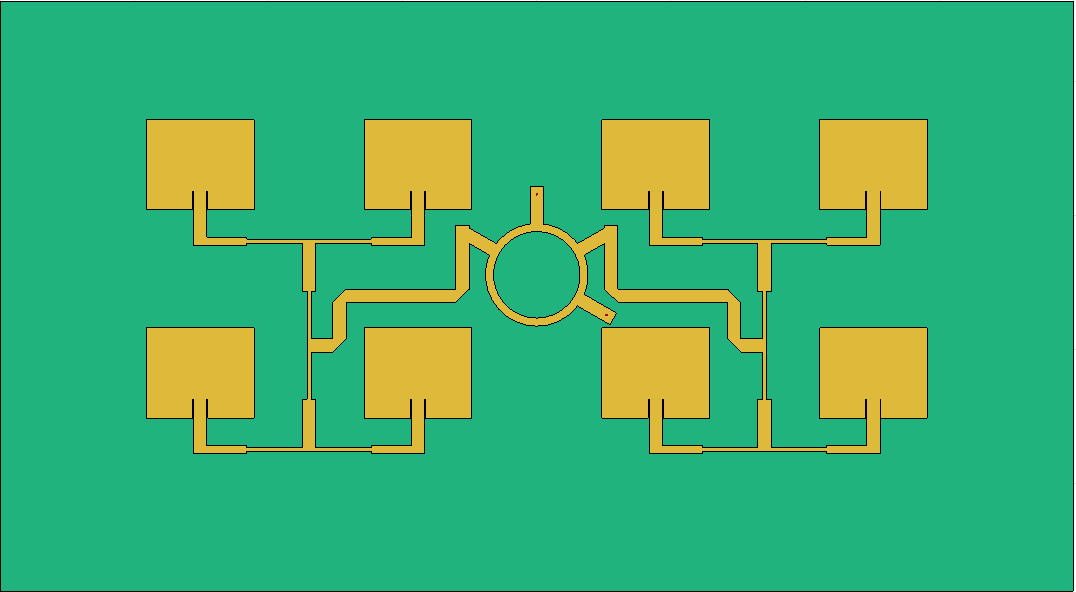Create Antenna Array Geometry

Use `couplerRatrace `object and assign appropriate values to the properties such that it resonates at 3 GHz.

```%create RatRace by assiging the values to couplerRatrace component %create RatRace cup = couplerRatrace; d = cup.Substrate; cup.PortLineLength = 18.6e-3; cup.PortLineWidth = 0.0050; cup.CouplerLineWidth = 0.0030; cup.Circumference = 0.111;```

Use the `pcbComponent `object to form a pcb stack of the Rat-race coupler such that the ground plane can be extended to accommodate the patch antenna array.

```RR = pcbComponent(cup); RR.BoardShape = antenna.Rectangle("Center",[0 0.02],"Length",0.4,"Width",0.22); RR.Layers{3} = RR.BoardShape; ground = RR.BoardShape;```

Re-alignment of the ports of the Rat-race coupler is required to arrange the ratrace coupler at the center of the patch antennas such that the distance betwen the patch antennas on both sides is same. Use `antenna.Rectangle `to create the the rectangle shapes and use Boolean add and subtract operations to modify the Rat-race coupler as required in this design. Use `show` method to visualize the structure.

```top = RR.Layers{1}; cut1 = antenna.Rectangle("Center",[34.4e-3 0e-3],"Length",3e-3,"Width",5e-3); cut2 = antenna.Rectangle("Center",[-34.4e-3 0e-3],"Length",3e-3,"Width",5e-3); top = top-cut1-cut2; top = rotateZ(top,-30); cutfifine1 = antenna.Rectangle("Center", [0e-3,35.9e-3],"Length",7e-3,"Width",6e-3); top = top-cutfifine1; top = rotateZ(top,-30); cutfifine2 = antenna.Rectangle("Center",[35.9e-3,0],"Length",6e-3,"Width",7e-3); top = top-cutfifine2; top = rotateZ(top,30); top = translate(top,[0,28e-3,0]); figure show(top);```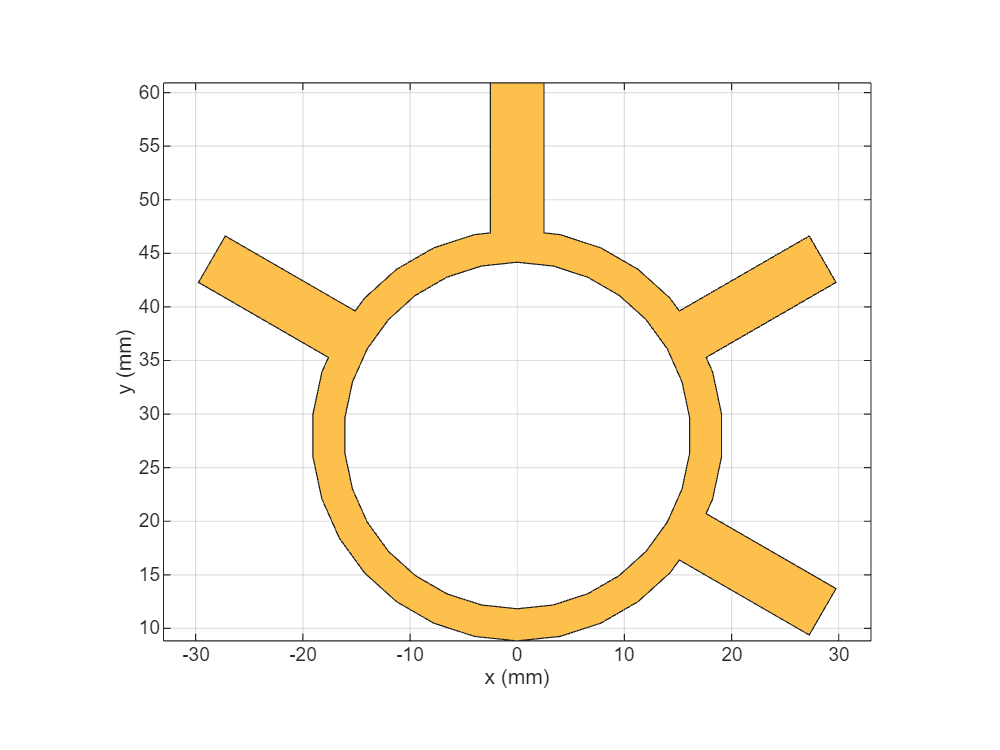#### Create patch antenna

Use `patchMicrostripInsetfed `object to create the patch antenna array. Assign the values for the properties of the antenna such that it resonates at 3 GHz.

```ant = patchMicrostripInsetfed; ant.Length = 33.6309e-3; ant.Width = 40.1597e-3; ant.Height = 1.6e-3; ant.Substrate = d; ant.NotchLength = 7e-3; ant.GroundPlaneLength = 0.0600```
```ant = patchMicrostripInsetfed with properties: Length: 0.0336 Width: 0.0402 Height: 0.0016 Substrate: [1x1 dielectric] PatchCenterOffset: [0 0] FeedOffset: [-0.0300 0] StripLineWidth: 1.0000e-03 NotchLength: 0.0070 NotchWidth: 0.0030 GroundPlaneLength: 0.0600 GroundPlaneWidth: 0.0600 Conductor: [1x1 metal] Tilt: 0 TiltAxis: [1 0 0] Load: [1x1 lumpedElement] ```
```ant.StripLineWidth = 5e-3; ant.NotchWidth = 5.577e-3;```

#### Create antenna array

Use `pcbStack` to convert the antenna into a PCB stack and copy the designed antenna element to create 8 similar elements. Use `translate` function to position the patch antennas on both the sides of the Rat-race coupler to create the a 2-by-4 array antenna elements and visualize it using the `show` function.

```array = pcbStack(ant); a1 = array.Layers{1}; a1 = rotateZ(a1,90); a2 = copy(a1); a3 = copy(a1); a4 = copy(a1); ant1 = translate(a1,[-125.5202e-3,69e-3,0]); ant2 = translate(a2,[-125.5202e-3,-0.008650,0]); ant3 = translate(a3,[-44.3591e-3,69e-3,0]); ant4 = translate(a4,[-44.3591e-3,-0.008650,0]); antArrayleft = ant1+ant3+ant2+ant4; antArrayright = copy(antArrayleft)```
```antArrayright = Polygon with properties: Name: 'mypolygon' Vertices: [331x3 double] ```
```antArrayright = mirrorY(antArrayright); antArray = antArrayleft+antArrayright; show(antArray);```Use `antenna.Rectangle to c`reate a part of the power divider network. Create the power divider using `traceTee` shape . Use `copy `and `mirrorX` operation to create 4 power dividers with same dimensions.

```inline1 = antenna.Rectangle("Center",[-54.29e-3,0.0405],"Length",15e-3,"Width",2.9e-3); inline2= antenna.Rectangle("Center",[-115.6e-3,0.0405],"Length",15e-3,"Width",2.9e-3); inline3 = antenna.Rectangle("Center",[-54.29e-3,-37.1475e-3],"Length",15e-3,"Width",2.9e-3); inline4 = antenna.Rectangle("Center",[-115.6e-3,-37.1475e-3],"Length",15e-3,"Width",2.9e-3); tee = traceTee; tee.Length = [46.3746e-3 18e-3]; tee.Width = [1.492e-3,5e-3]; tee1 = copy(tee); tee2 = copy(tee); tee3 = copy(tee); tee.ReferencePoint = [84.92e-3,0.0405]; tee2 = mirrorX(tee2); tee3 = mirrorX(tee3); tee1.ReferencePoint = [-84.92e-3,0.0405]; tee2.ReferencePoint = [84.92e-3,37.1475e-3]; tee3.ReferencePoint = [-84.92e-3,37.1475e-3]; teecut = antenna.Circle("Center",[12e-3,12e-3],"Radius",3e-3,NumPoints=3); ytee = traceTee; ytee.Length = [45.3746e-3 9.12456e-3]; ytee.Width = [1.492e-3,5e-3]; y1tee = copy(ytee); ytee.ReferencePoint = [-1.675e-3,84.92e-3]; ytee = rotateZ(ytee,-90); y1tee.ReferencePoint = [1.675e-3,84.92e-3]; y1tee = rotateZ(y1tee,90); fifine = antenna.Rectangle("Center", [-73.585e-3,9.175e-3],"Length",5e-3,"Width",20e-3); fifine1 = antenna.Rectangle("Center", [-52.57e-3,20.105e-3],"Length",47e-3,"Width",5e-3); fifine2 = antenna.Rectangle("Center", [-27.7e-3,32.6e-3],"Length",5e-3,"Width",30e-3); curve = antenna.Polygon('Vertices',[-84.085e-3 -0.825e-3 0;-79.085e-3 -0.825e-3 0; ... -79.085e-3 4.175e-3 0]); curve = translate(curve,[8e-3,0,0]); curve1 = antenna.Polygon('Vertices',[-84.085e-3 22.705e-3 0;-84.085e-3 17.605e-3 0; ... -79.085e-3 22.705e-3 0]); curve1 = translate(curve1,[8e-3,0,0]); curve2 = antenna.Polygon('Vertices',[-33.2e-3 17.6e-3 0;-33.2e-3 22.605e-3 0; ... -38.4e-3 17.6e-3 0]); curve2=translate(curve2,[8e-3,0,0]); sqcut = antenna.Rectangle("Center", [-26.7e-3,47.518e-3],"Length",7e-3,"Width",2.1640e-3); sqcut2 = copy(sqcut); sqcut2 = mirrorY(sqcut2); RRline = fifine+fifine1+fifine2-curve-curve1-curve2; feedLeft = tee1+tee3+inline1+inline2+inline3+inline4+y1tee+RRline; feedRight = copy(feedLeft); feedRight = mirrorY(feedRight); totaltop = top+feedLeft+feedRight+antArray-sqcut-sqcut2;```

#### Create PCB antenna from geometry

Use the `pcbStack` object to create a stack of the layers of the antenna and assign the shape created in the previous section to the top layer of the `pcbStack`. Thus the design of the patch antenna is complete.

```RRmicrostrip = pcbStack; RRmicrostrip.Layers{1} = totaltop; RRmicrostrip.BoardThickness = 0.0016; RRmicrostrip.BoardShape = antenna.Rectangle("Center",[0 0.02],"Length",0.4,"Width",0.22); RRmicrostrip.Layers = {totaltop,d,ground}; feedloc = [[0 58e-3 3 1];[26e-3 13e-3 3 1]]; RRmicrostrip.FeedLocations = feedloc; RRmicrostrip.FeedDiameter = 0.005/8; RRmicrostrip.FeedViaModel = 'strip'; RRmicrostrip.FeedPhase = 0; show(RRmicrostrip )```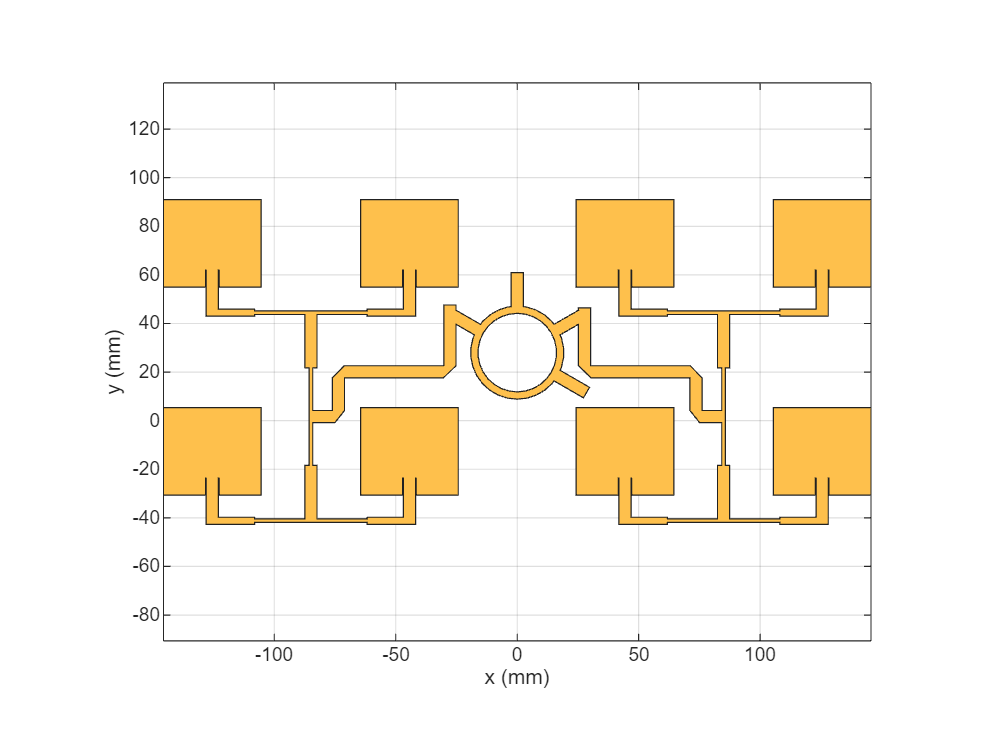Analyze the Antenna

Set the `FeedVoltage` property of `pcbStack` to [1 0] to feed a single port amongst two.

```%To get the sum port characteristics RRmicrostrip.FeedVoltage = [1 0]; %To get the Difference port characteristics %RRmicrostrip.FeedVoltage = [0 1];```

Use `mesh` function to manually mesh the array antenna. Use `MaxEdgeLength` equal to `lambda`/8 times the center frequency to get a finer mesh where` lambda `represents the wavelength.

```plotfrequency = 3.3*1e9; lambda = 3e8/plotfrequency; mesh(RRmicrostrip,'MaxEdgeLength',lambda/8);```Estimate memory requirement

Use the `memoryEstimate` function to calculate the memory required to solve the structure.

`memEst = memoryEstimate(RRmicrostrip,3e9);`

Calculate S`-`parameters

Use the `sparameters` function to calculate the S-parameters.

```frequencyRange = (2.5:0.1:3.5)*1e9; %s = sparameters(RRmicrostrip,frequencyRange); %rfplot(s)```

To save simulation time, the code for calculating and plotting the s-parameters is commented out and a pre-computed result is provided in the` RRmicrostripdata.mat `file. You can uncomment the code and use the freshly computed results instead of the MAT-file for the analysis.

Load the `RRmicrostripdata.mat `file. It contains the simulated and measured data stored in four variables namely `S11_simulated`, `S11_measured`, `patternsum_simulated`, `patternsum_measured, patterndiff_simulated and patterndiff_measured `. Use `S11_simulated `to plot the s-parameters.

```load sBandmonopulse.mat figure; plot(simufreq,S11_simulated,'r',LineWidth=2); hold on; plot(simufreq,S12_simulated,'b',LineWidth=2); hold off; legend('S11','S12'); xlabel('Frequency (MHz)'); ylabel('Reflectioncoefficient'); ```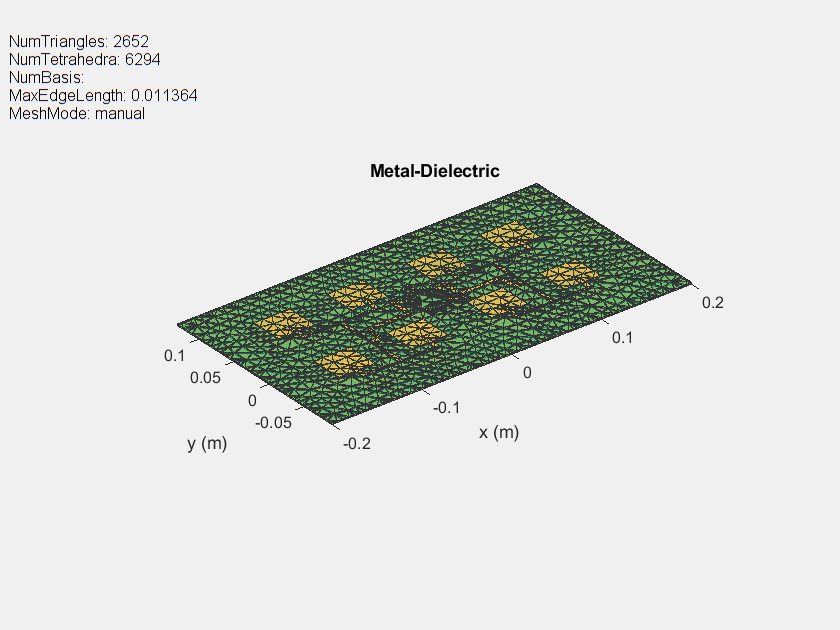Use the `pattern` function to plot the 3D ration Pattern at 3.3GHz.

```figure; pattern(RRmicrostrip,3.3e9);```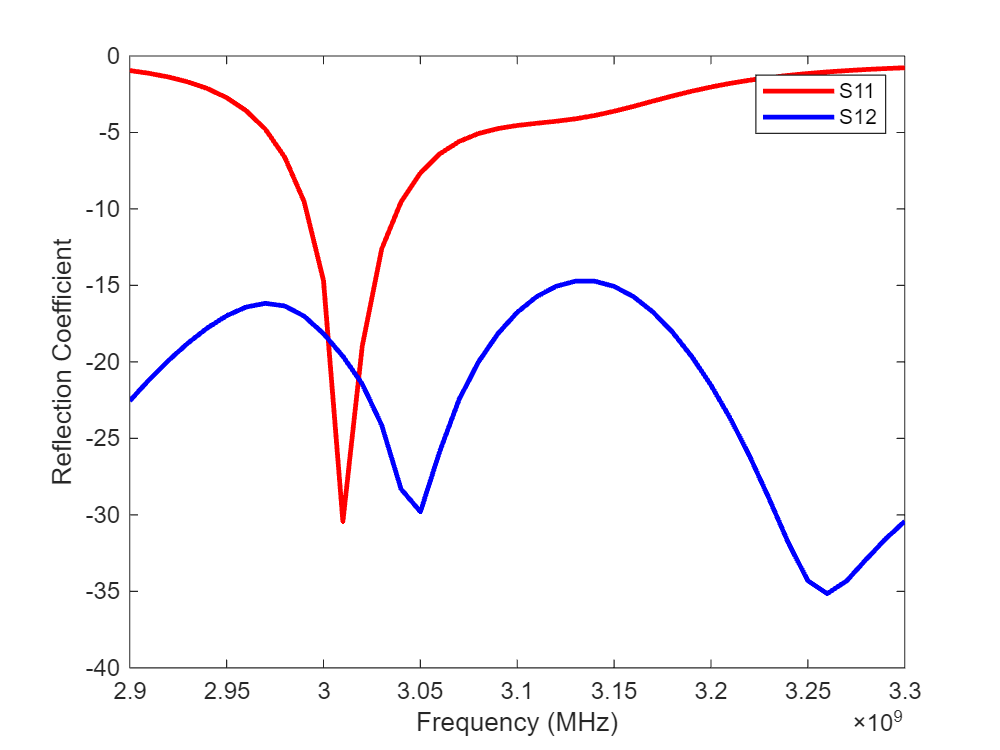Use the` Pattern` function to plot the 2D radiation pattern at an elevation angle of 0 deg`.`

```figure; pattern(RRmicrostrip,plotfrequency,0,0:1:360);```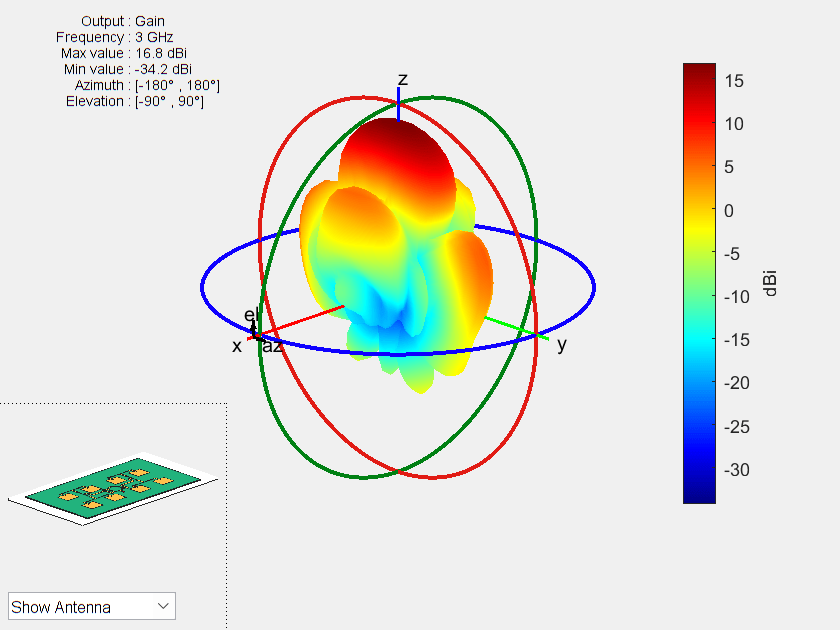The designed array antenna is fabricated as shown below and it is tested for the reflection coefficient and the radiation pattern at both sum and difference ports. Reflection coefficient of fabricated array antenna is measured on Network Analyzer. Radiation pattern measurements are performed at an anechoic chamber.

Top view of the fabricated antenna is shown below.### Measured results

Create the comparison graph for measured reflection coefficient.

``` plot(measfreq,S11_measured','b',LineWidth=2); xlabel('Frequency (MHz)'); ylabel('Reflectioncoefficient'); ```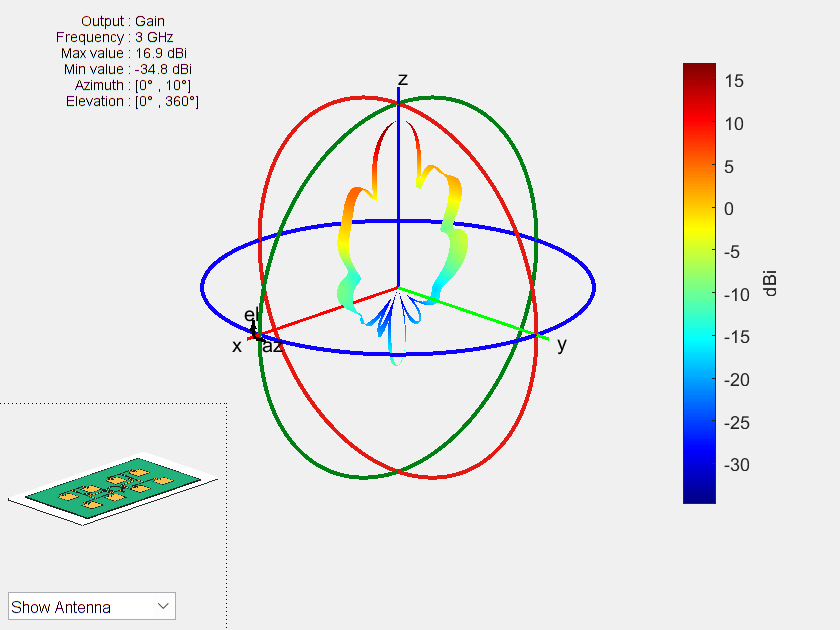Create the graph for measured sum radiation pattern.

``` plot(-180:10:180,sumpattern_measured','b',LineWidth=2); xlabel('Theta(degree)'); ylabel('NormalizedGain'); ```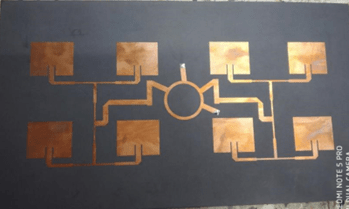Create the graph for measured diffrence radiation pattern.

```plot(-44:2:46,diffpattern_measured,'r',LineWidth=2); xlabel('Theta(degree)'); ylabel('NormalizedGain');```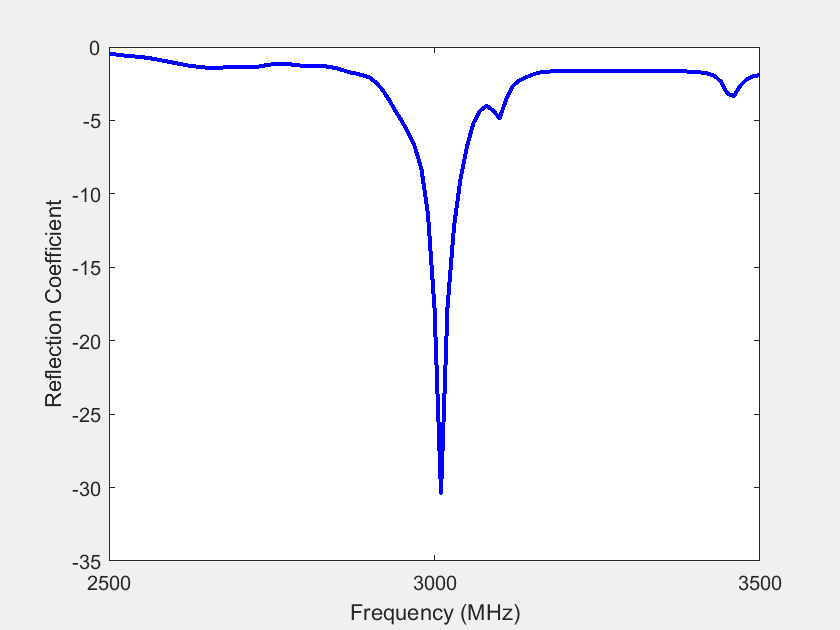Conclusion

The designed antenna has a maximum gain of 15.3 dB sum port gain and 20 dB null depth at difference port at 3GHz. The efficiency of the designed array antenna is confirmed by measurements. The measured and simulated results agree well. The designed array antenna is a promising candidate for monopulse tracking radar at S-band.

Reference

 H. Kumar and G. Kumar, "Microstrip antenna array with ratrace comparator at X-band for monopulse tracking radar," 2016 IEEE International Symposium on Antennas and Propagation (APSURSI), 2016, pp. 513-514, doi: 10.1109/APS.2016.7695965.